# Finite Field Arithmetic

When you were six years old, you probably spent quite a bit of time reciting multiplication tables and doing long additions and multiplications. You might have found it hard to remember to pass the carry from one column of digits to the next in an addition, but you did it because the teacher said, "This is the way the world works." Now you encounter finite field arithmetic, which has a different set of rules and, on first inspection, sounds like it came from outer space. Finite field arithmetic is important in cryptography and is the basis of the familiar cyclic redundancy check (CRC) used to detect errors in data packets.

Conventional arithmetic operates on an infinite range of values, even if you limit it to positive integers. However, if your entire universe is defined by a single byte, you have only 256 values to deal with. What often happens is that normal arithmetic is applied to byte values and any overflows or underflows during the conventional arithmetic are discarded. This works for many types of calculations, but in some sense discarding the carry violates the rules of conventional arithmetic. Your primary school teacher didn't talk about number universes that had only 256 values. Finite field arithmetic is defined specifically to handle such finite number universes. The rules apply to cases like single byte arithmetic so, in some sense, it is more valid than the familiar arithmetic. But let's not get too philosophical here; this type of arithmetic enables some good tricks and allows some neat shortcuts in the computations. This section is not intended to be a rigorous description of finite field mathematics; and if you are a pure mathematician, you probably won't like it. However, we do introduce the basics and explain why some of the computations used in cryptography look a little weird.

For our application, we are interested only in finite fields that can be represented by binary numbers. For purposes of finite field arithmetic, we can represent a binary number by polynomials of the form:

Equation A.1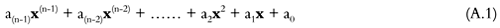The value of x is not important as it is only the coefficients a(n) that we are interested in. However if the coefficients have the value 0 or 1 and x represents the value 2, then the value of the polynomial, computed using conventional arithmetic, corresponds to the binary value.

Each of the coefficients corresponds to one bit of a binary number. So, for example, the 8-bit value 10010111 would be written as:

Equation A.2or more simply:

Equation A.3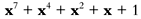Treating the numbers as polynomials leads to some interesting and different behavior when you are performing arithmetic operations. This is okay, providing such treatment still follows some basic rules such as:

if A + B = C then A = B C

and if A x B = C then A = C÷B

In the following sections we look at the main operations in turn.

When you add two polynomials, each term is added independently; there is no concept of a carry from one term to another. For example, in conventional arithmetic

Equation A.4In our binary representation, the coefficients can be only 0 or 1. The value 2 is not possible. Therefore, we have the rule that, when adding the coefficients, the following addition rule applies:

• 0 + 0 = 0

• 0 + 1 = 1

• 1 + 0 = 1

• 1 + 1 = 0 (there is no carry)

By a useful coincidence, this is the same result as what you get when you perform an exclusive OR operation, which is easier for digital logic than a binary addition.

Using our binary rules, the addition of the two polynomials in equation A.4 is:

Equation A.5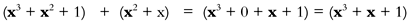This corresponds to the binary computation:

Equation A.6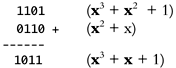Notice how the addition has now been entirely replaced by the exclusive OR operation. Addition of 2 bytes under these rules is really an XOR operation, and addition cannot have a result bigger than 1 byte, which is consistent with our 1-byte universe.

#### Subtraction

The same logic that made addition become XOR also applies to subtraction. Suppose we want to subtract the two polynomials in (A.4). In conventional arithmetic the result would be:

Equation A.7The coefficient of the x term is 1. There is no 1 value in a single binary digit. The subtraction table for two binary digits is:

• 1 1 = 0

• 1 0 = 1

• 0 1 = 1 (there is no borrow)

• 0 0 = 0

Once again this is the same as the XOR operation so that the binary subtraction takes the form: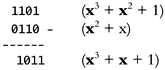Surprising but true in this byte arithmetic universe addition and subtraction become the same operation and are replaced by the exclusive OR operation. Notice also how this new arithmetic also obeys the rule that if A + B = C, then A = B C.

#### Multiplication

Multiplication deviates even more from conventional arithmetic because of the way polynomials multiply together. The basic rule for multiplying two polynomials is to multiply all the terms together and then add terms of similar order (in other words, the same power of x). Here is a simple example in normal mathematics:

Equation A.7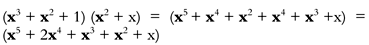By now you might guess that in our binary universe the x4 term will disappear, leading to the result:

Equation A.8So far this looks straightforward enough and, by following the good old school long multiplication rules, we can work out the multiplications using only shift and XOR operations:

Equation A.9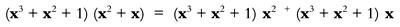Multiplying by x2 is the same as shifting left by two places because:

Equation A.10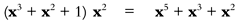This means that the long multiplication can be done using the accumulate row method as follows:

Equation A.11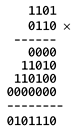Notice how the intermediate rows are just the first value shifted and the result is just the XOR of the rows. Great! Now we have a really efficient way to do addition, subtraction, and multiplication by using this polynomial-based arithmetic. However, there is a snag that we discuss after looking at division.

#### Division

Division works by the shift and subtract method familiar under the name long division. Of course, in our case the subtraction is done using an XOR operation. An example is shown here and should be fairly self-explanatory:

Equation A.12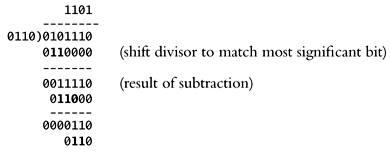This is the reverse of the multiplication shown in the previous section. Gratifyingly, we get back to the result before the multiplication, showing that our arithmetic satisfies the rules that if A x B = C, then B = C ÷ A.

#### Galois Field GF()

Now comes the hard part. Not hard to implement but hard to understand. When we did addition and subtraction, it was not possible for the result to overflow. The result always fitted into a byte. However, based on our long multiplication approach, it is clearly possible that the result of multiplying two 8-bit numbers could be more than 8 bits long. Such an overflow is not allowed to exist in our finite field of 256 values, so what has gone wrong?

Let's go back to ordinary numbers instead of polynomials for a moment. Let's define a finite field that comprises seven digits {0, 1, 2, 3, 4, 5, 6}. We will define addition and multiplication to be the conventional operations except that the result "rolls over" from 6 back to 0. So if we add 1 to 6, we get 0. If we multiply 2 by 4, we get 1 (2 * 4 = 4 + 4 = 6 + 2 = 1 work it out). We can make an addition table, as shown in Table A.1. Taking any two numbers from the top row and left column, the sum is found in the intersection of the row and columns.

0

1

2

3

4

5

6

0

0

1

2

3

4

5

6

1

1

2

3

4

5

6

0

2

2

3

4

5

6

0

1

3

3

4

5

6

0

1

2

4

4

5

6

0

1

2

3

5

5

6

0

1

2

3

4

6

6

0

1

2

3

4

5

##### Table A.2. Multiplication Table

0

1

2

3

4

5

6

0

0

0

0

0

0

0

0

1

0

1

2

3

4

5

6

2

0

2

4

6

1

3

5

3

0

3

6

2

5

1

4

4

0

4

1

5

2

6

3

5

0

5

3

1

6

4

2

6

0

6

5

4

3

2

1

In a similar way we can define a multiplication table, as shown in Table A.2. Here the product value is in the intersection of each row and column. These tables can be used for subtraction and division as well. To work out the value of m/n, you go to the nth column, find the entry m in the column, and the answer is the row number. For example, to compute 6/3, go to the third column and find the value 6, then look to the left to see the answer 6/3 = 2. Note that this rule does not work if you try to divide by 0; try it and you'll see the problem. As with conventional arithmetic, dividing by 0 is undefined.

These tables show that it is possible to define a finite number universe with familiar and useful arithmetic operations that really work. However, this approach does not work in all cases. Suppose we want a universe with six numbers: {0, 1, 2, 3, 4, 5}. Applying the "roll over rule" whereby 5 + 1 = 0, the addition and multiplication tables come out as shown in Tables A.3 and A.4.

Look at column 2 of the multiplication table. 0 appears twice. Numbers 1, 3, 5 don't appear at all! Column 3 only has the values 0 and 3. What this means is that it is impossible to do meaningful division in this number universe. The problem is that there are six numbers and both 2 and 3 are factors of 6. This means that 2 * 3 = 0 and also 2 * 0 = 0.

0

1

2

3

4

5

0

0

1

2

3

4

5

1

1

2

3

4

5

0

2

2

3

4

5

0

1

3

3

4

5

0

1

2

4

4

5

0

1

2

3

5

5

0

1

2

3

4

##### Table A.4. Multiplication Table

0

1

2

3

4

5

0

0

0

0

0

0

0

1

0

1

2

3

4

5

2

0

2

4

0

2

4

3

0

3

0

3

0

3

4

0

4

2

0

4

2

5

0

5

4

3

2

1

Because of this factoring problem, this type of finite number universe can only have all four arithmetic operations (+, , *, ÷) if the number of values in the universe is a prime number. It works when there are seven values because seven is a prime number. It doesn't work with eight, nine, or ten values, but it works with eleven values and so on.

If we return to our polynomial representation, a similar rule applies. The finite field should be bounded by a polynomial that is irreducible. A polynomial is reducible if it can be factored. For example (x2 + 1) is reducible because:

Equation A.13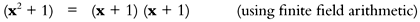To make our finite field arithmetic work, we need a finite field that is bounded by an irreducible polynomial and has 256 elements. Such a field can be created and is called a Galois Field, denoted by GF(256). We will not present the theory behind how a Galois Field is created. However, it has the property that it has 2n entries and the entries are derived from, and bounded by, an irreducible polynomial. For GF(256), that polynomial is:

Equation A.14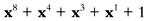This corresponds to the binary value 100011011 or hexadecimal 11B.

Let's remind ourselves of why we digressed to discuss the Galois Field. The problem was that multiplication according to the rules we had defined caused undefined results due to overflows. The question was how these should be handled and avoided? The answer lies in treating the possible 256 values as members of the GF(256) field. This field is limited by the irreducible polynomial that defines our GF(256). We can think of the rules of multiplication in the same way as shown in Table A.2. In that case the result wraps around the prime number that defines the number of elements in the field; in Table A.2 this prime number is 7. In conventional arithmetic, we would say that the result is the remainder after dividing by 7 or that the result is computed modulo 7.

In Table A.2, a multiplication can be computed as follows:

Result = (A x B) mod 7.

or

Result = Remainder((A x B) / 7)

Example: 6 * 6 = 36 mod 7 = Remainder(36/7) = 1

The same rule is now applied to our byte computations in the GF(256) field:

Result = (A x B) mod x8 + x4 + x3 + x1 + 1 (the irreducible polynomial)

or

Result = Remainder( (A x B) / (x8 + x4 + x3 + x1 + 1) )

Lets see how this works in practice. We saw in (A.11) the result of a multiplication that did not overflow outside the byte value. Let's take an example that clearly does want to overflow:

` 01101001 * 00101001 `

Using the accumulate row approach:

Equation A.15The intermediate result in (A.15) is 12 bits long we need to reduce it by wrapping around the irreducible polynomial. To do this, we need to divide by our irreducible polynomial and take the remainder:

Equation A.16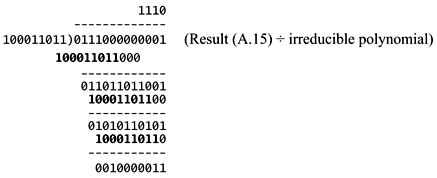So the remainder after removing the overflow is 10000011. In other words, in our finite field:

` 01101001 * 00101001  =  10000011 `

All values are within our single byte space. Hooray! However, although it still uses only shift and XOR, the computation now seems rather complicated and long-winded. We had to do both long multiplication and long division to get the result. There is a good trick we can use to simplify the computation, however. If we look at the accumulate row approach to multiplication, each intermediate row represents the first multiplier shifted left to correspond to a bit in the second multiplier. After this, all the rows are added together and the result is taken modulus our field polynomial 100011011. However, rather than waiting until all the rows are accumulated before adding together, you can shift and add 1 bit at a time as the computation proceeds. Let's look at the previous multiplication, 01101001 * 00101001, done in this way. The sequence of events is shown in Figure A.1. The process for computing A x B is as follows:

• Take each bit of B in turn, starting with the most significant bit.

• For each bit, first multiply the accumulator by 2 (shift left).

• If the bit is 1, add the value of A to the accumulator.

##### Figure A.1. Multiply by Shift and AddThe final value of the accumulator is the answer.

Figure A.1 shows the computation for the same example we used earlier (A.15) and you can see that the result is the same this is good. In the previous example we reduced the result of (A.15) to be within range by dividing by the value 100011101 and taking the remainder. This gave the result shown in (A.16). However, if we use the shift and add approach, there is another way to do the reduction that is easier than performing this long division. Note that the shift and add method requires that the accumulator be shifted left at each stage. This left shift is the same as multiplying by two. The simplification is to reduce the value within range after each shift rather than waiting until the end.

You might think that means more divisions rather than fewer. However, consider the result of the computation 2 * A. If the most significant bit of A is 0, we know that there cannot be an overflow so the result will already be in range. If the most significant bit is 1, we know that there will be an overflow. Because we know the result overflows, it needs to be reduced to get back to a byte value. We also know that the range of possible values after the shift will be 100000000 to 111111110. This means that the result of dividing by 100011011 must always be 1 and the remainder will be the result we want. Because we know the result of the division is 1, we can get that wanted remainder simply by subtracting 100011011 from the shifted value. So now we have a simple rule for computing 2 * A:

Equation A.17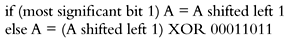In the second case, the XOR accomplished the "subtract 100011011" operation for the byte value.

Now we have a long multiplication rule that works for all cases. The shift operation in Figure A.8 is replaced by the formula in (A.17). Each intermediate step as well as the final result is guaranteed to be within the GF(256) field; in other words, the result of the multiplication is always a single byte. The long multiplication has been achieved by a short sequence of XOR operations and shifts that are easily implemented in digital systems.

#### Conclusion

This section introduces mathematics that may be unfamiliar to you. The arguments seem logical, but you may be left feeling that the operations are puzzling and nonintuitive. However, the benefit of this type of mathematics is an amazing simplification in the way multiplication is implemented. It makes the design and implementation of encryption systems, which often rely on many multiplications, much more practical in the real world.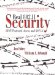Real 802.11 Security: Wi-Fi Protected Access and 802.11i
ISBN: 0321136209
EAN: 2147483647
Year: 2005
Pages: 151